# 集算器 - 敏捷计算编程语言

## 集算器是什么

• 一种面向结构化数据的程序计算语言
• 集算器又称：SPL（Structured Process Language）
• 敏捷计算是集算器的主要特征• 只能实施规整计算
• 无法完成数据准备

• 只能处理模式化的计算
• 无法完成灵活的复杂计算

• 可以处理所有情况

## 为什么是SPL

### SQL的不足

SQL作为常使用的结构化数据处理语言存在如下问题

##### 难写难调试

SQL不提倡过程，难以实现复杂计算，经常要写得很长，难写难调试

##### 过于封闭

SQL强依赖数据库，无法计算文件等库外数据，很难跨库计算

### SQL与SPL对比

```select max(continuousDays)-1
from (select count(*) continuousDays
from (select sum(changeSign) over(order by tradeDate) unRiseDays
case when closePrice>lag(closePrice) over(order by tradeDate)
then 0 else 1 end changeSign
from stock) )
group by unRiseDays)```

#### SQL解法

SQL在使用窗口函数的情况下嵌套三层完成；

A
2 =0
3 =A1.max(A2=if(closePrice>closePrice[-1],A2+1,0))

### Python的不足

Python相对SQL更加灵活开放，但仍存在如下问题

##### 难以实现复杂计算

pandas不是专业结构化数据计算包，在处理分组有序等复杂运算时较为繁琐

##### 使用门槛太高

pandas等计算包只有专业程序员才能玩得转

### Python与SPL对比

```import time
import numpy as np
import pandas as pd
s = time.time()
sales['ORDERDATE']=pd.to_datetime(sales['ORDERDATE'])
sales['y']=sales['ORDERDATE'].dt.year
sales['m']=sales['ORDERDATE'].dt.month
sales_g = sales[['y','m','AMOUNT']].groupby(by=['y','m'],as_index=False)
amount_df = sales_g.sum().sort_values(['m','y'])
yoy = np.zeros(amount_df.values.shape)
yoy=(amount_df['AMOUNT']-amount_df['AMOUNT'].shift(1))/amount_df['AMOUNT'].shift(1)
yoy[amount_df['m'].shift(1)!=amount_df['m']]=np.nan
amount_df['yoy']=yoy
print(amount_df)
e = time.time()
print(e-s)```

#### Python解法

Python通过多步算出结果，实现起来比SQL更容易

A
1 =file("C:\\sales.csv").import@t()
2 =A1.groups(year(ORDERDATE):y,month(ORDERDATE):m,sum(AMOUNT):x)
3 =A2.sort(m)
4 =A3.derive(if(m==m[-1],x/x[-1]-1,null):yoy)

#### SPL解法

SPL的分步代码更为简洁，实现更为简单

### 其他现有编程语言的不足JAVA和VBA都不是为结构化数据计算设计的，缺少集合化计算类库，编码过于复杂

SPL才是处理结构化数据简洁有效的编程语言

### 简洁易用的开发环境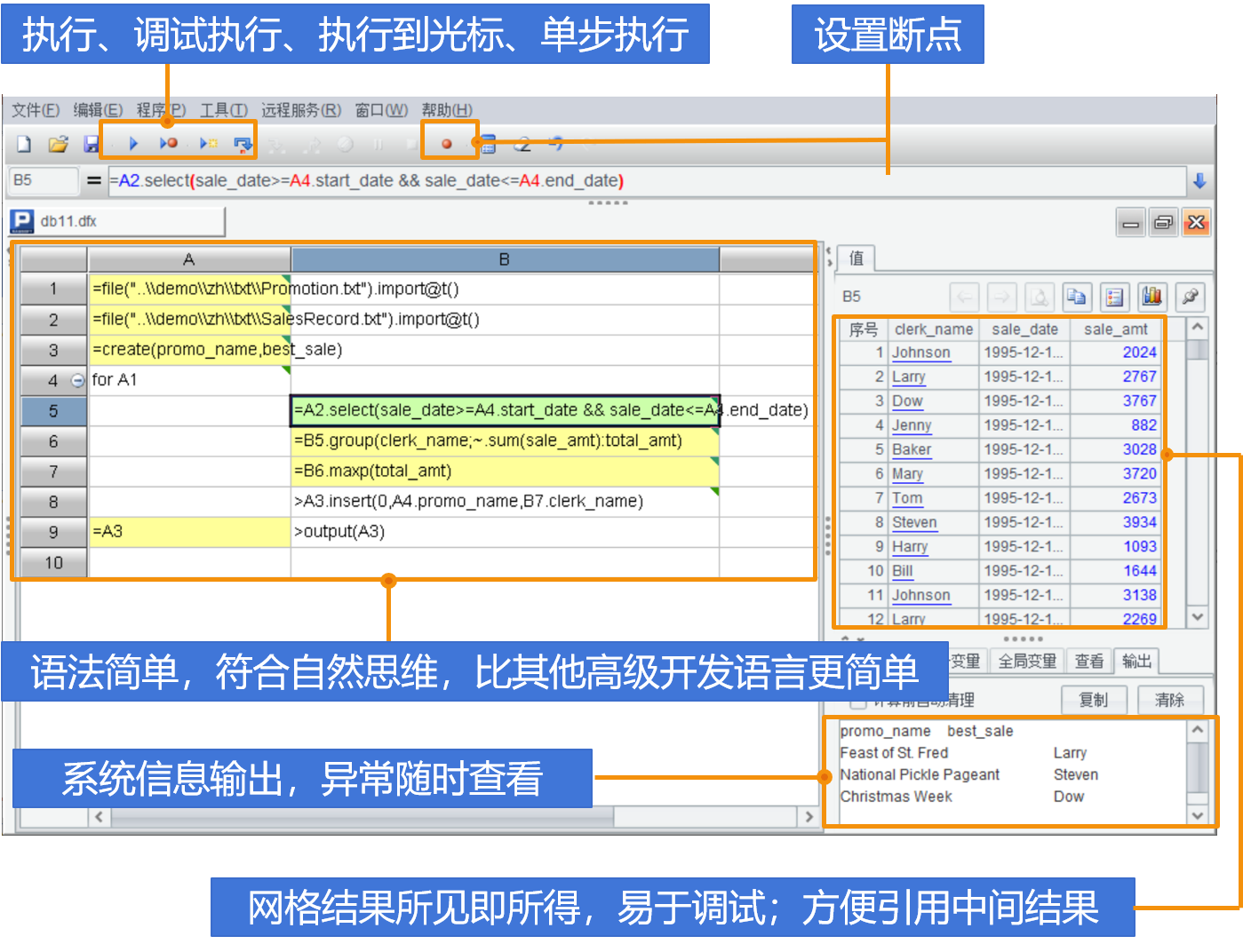### 专门设计的语法体系

SPL特别适合复杂过程运算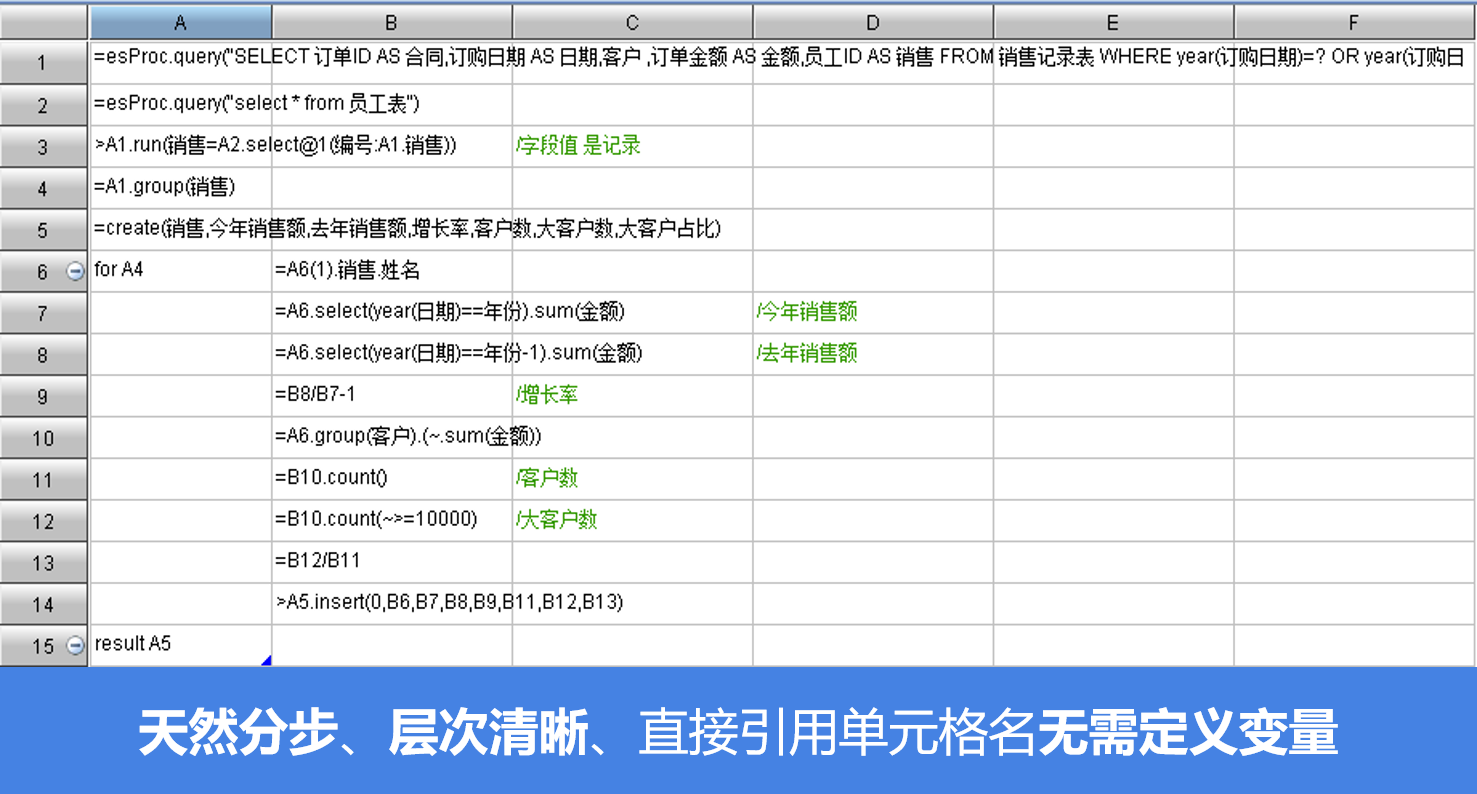### 丰富的运算类库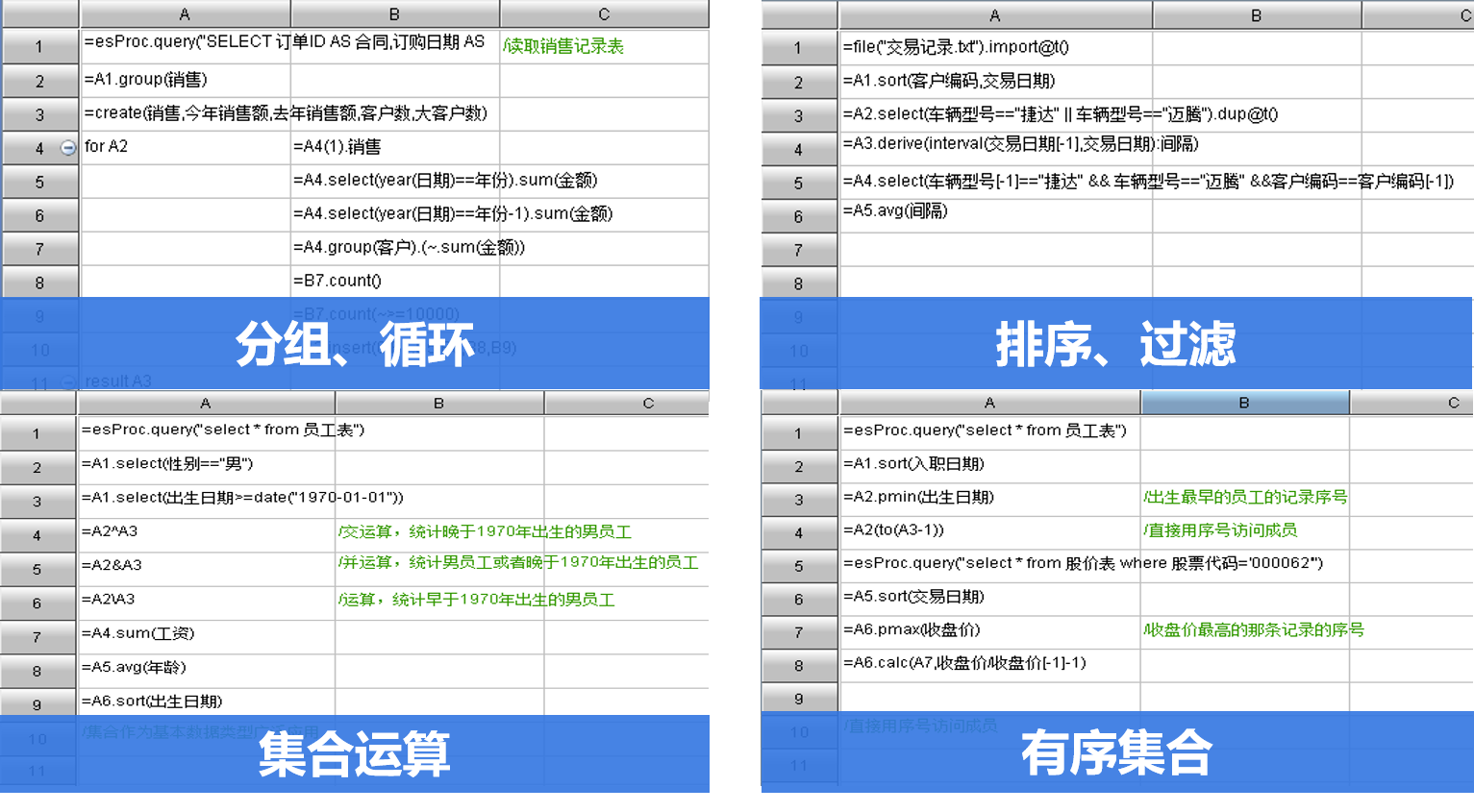## SPL语言理念

• 无法描述集合的集合
• 字段取值不能再是集合

### 离散性

#### SQL缺乏离散性

• 没有天然序号，引用集合指定成员很麻烦
• 只有单行记录的表，没有游离记录

### 彻底的集合化

#### 显式集合数据类型

A
1 =人员表.group(生日).select(~.count(1)>1).conj()

#### 集合运算

A
1 =V.len()
2 =V.to(A1\4+1,A1*3\4).run(value-=V(A1\2).value)

#### 成员再构成集合进行运算

A B
1 ...
2 =A1.select(height>=1.7) =A1.select(sex=="Female")
3 =A2^B2 =A1.select(height>=1.7&&sex=="Female")
4 =A2\B2 =A1.select(height>=1.7&&sex!="Female")

### 强Lambda语法解决有序运算

#### 本值引用~

A B
1 ... ...
2 =A1.select(sex=="Male") =A1.select(sex=="Female")
3 =A2.conj(B2.([A2.~,~])) =A3.minp@a(abs(~(1).height-~(2).height))

#### 序号引用#

A
1 ...
2 =A1.group((#+2)\3)
3 =A1.group(#%3+1)

#### 相邻成员与集合引用

A
... ...
3 =A2.derive(price-price[-1]:gain)
4 =A2.derive(price[-1:1].avg():mavg)

### 举例：返回集合的聚合

#### 计算任务：列出每个用户最近一次登录间隔

```WITH TT AS
(SELECT RANK() OVER(PARTITION BY uid ORDER BY logtime DESC) rk, T.*  FROM 登录表 T)
SELECT uid,(SELECT TT.logtime FROM TT where TT.uid=TTT.uid and TT.rk=1)
-(SELET TT.logtim FROM TT WHERE TT.uid=TTT.uid and TT.rk=2) 间隔
FROM 登录表 TTT GROUP BY uid ```

A
1 =登录表.groups(uid;top(2,-logtime)) 最后2个登录记录
2 =A1.new(uid,#2(1).logtime-#2(2).logtime:间隔) 计算间隔

### 举例：跨行引用

#### 计算任务：计算每月前后各一个月的销售额移动平均值

```WITH B AS
(SELECT LAG(销售额) OVER (ORDER BY 月份) f1, LEAD(销售额) OVER (ORDER BY 月份) f2,   A.* FROM 销售表 A)
SELECT 月份,销售额,
(NVL(f1,0)+NVL(f2,0)+销售额)/(DECODE(f1,NULLl,0,1)+DECODE(f2,NULL,0,1)+1) 移动平均
FROM B
```

A
1 =销售表.sort(月份).derive(销售额[-1,1].avg()):移动平均)

### 举例：有序分组

#### 计算任务：一支股票最长连续上涨了多少天

```SELECT max(连续日数) FROM
(SELECT count(*) 连续日数 FROM
(SELECT SUM(涨跌标志) OVER ( ORDER BY 交易日) 不涨日数 FROM
(SELECT 交易日,
CASE WHEN  收盘价>LAG(收盘价) OVER( ORDER BY 交易日 THEN 0 ELSE 1 END 涨跌标志
FROM 股票 ))
GROUP BY 不涨日数)
```

A
1 =股票.sort(交易日).group@i(收盘价 < 收盘价[-1]).max(~.len())

### 举例：分组子集与有序分组混合

#### 计算任务：找出连续上涨三天的股票

```WITH A AS
(SELECT 代码,交易日, 收盘价-LAG(收盘价) OVER (PARITITION BY 代码 ORDER BY 涨幅) FROM 股票)
B AS
(SELECT 代码,
CASE WHEN 涨幅>0 AND
LAG(涨幅) OVER (PARTITION BY 代码 ORDER BY 交易日) >0  AND
LAG(涨幅,2) OVER PARTITION BY 代码 ORDER BY 交易日) >0
THEN 1 ELSE 0 END 三天连涨标志  FROM A)
SELECT distinct 代码 FROM B WHERE 三天连涨标志=1
```

A
1 =股票.sort(交易日).group(代码)
2 =A1.select((a=0,~.pselect(a=if(收盘价>收盘价[-1],a+1,0):3))>0).(代码)

### 举例：位置利用

#### 计算任务：某股票股价最高的那三天的平均涨幅

```SELECT AVG(涨幅) FROM
( SELECT 交易日, 收盘价-LAG(收盘价) OVER ( ORDER BY 交易日) 涨幅 FROM 股价表
WHERE 交易日 IN
(SELECT 交易日 FROM
(SELECT 交易日, ROW_NUMBER() OVER(ORDER BY 收盘价 DESC) 排名 FROM 股票)
WHERE 排名<=3 )
```

A
1 =股票.sort(交易日)
2 =A1.calc(A1.ptop(3,-收盘价),收盘价-收盘价[-1]).avg()

### 离散性支持下的集合运算

#### 合并汇总与拆分

• 列相同的表格合并
• 合并时去除重复的列
• 合并时汇总重复的列
• 横向拼接列不相同的表
• 行列方向都合并时去除重复项
• ……

• 查找某个值的位置
• 使用位置筛选
• 找到第一个或最后一个
• 找到最大最小值
• 选出前N名/后N名
• ……

#### 格值与汇总值计算

• 使用相邻行和区间计算
• 可能提前终止的累计
• 同类数据连续时使用同类相邻行计算
• 同类数据不连续时使用同类相邻行计算
• 使用同类数据的汇总信息
• …….

#### 集合运算和从属判断

• 简单成员的交并差
• 行式数据求交并差
• 不确定数量的集合求交并差
• 集合相等与从属判断
• 次序无关的集合相等与从属判断
• ……

#### 重复判断、计数与去除

• 判断是否有与自己重复的数据
• 统计重复次数
• 不确定多列一起统计重复次数
• 行式数据去重
• 简单数据去重
• …….

• 按指定次序对齐排列
• 指定次序有重复值的对齐
• 将并列排名的成员拼接起来
• 在相同分类内排序
• 分类下的排名
• ……

• 每N个成员分成一组
• 使用相邻数据作为分组条件
• 碰到空行或非空行时分组
• 按数据值的间隔分组
• 将分类内的数据拼接成文字
• ……

• 关联表引用
• 区间关联
• 二维关联表
• 使用区间范围倒查关联表
• 关联多行数据
• ……

#### 行列转换

• 固定列的行转列
• 行式表与交叉表互换
• 行列的高层分类互转
• 分类内数据横向拼入列
• 分类数据拼入列时要再分类或排序
• ……

• 生成连续的区间
• 根据数值将一行扩展出多行
• 拆分文字后扩展成多行
• 在连续值中补足缺失部分
• 每隔N行补足若干空行
• ……

## 集算器特色功能

### Excel配合

SPL与Excel配合可以增强Excel计算能力，降低计算实施难度

#### 使用剪贴板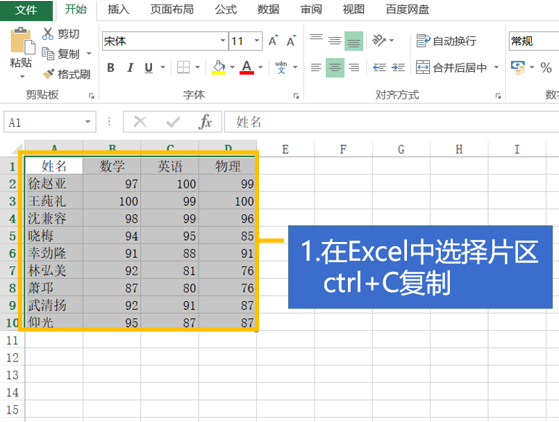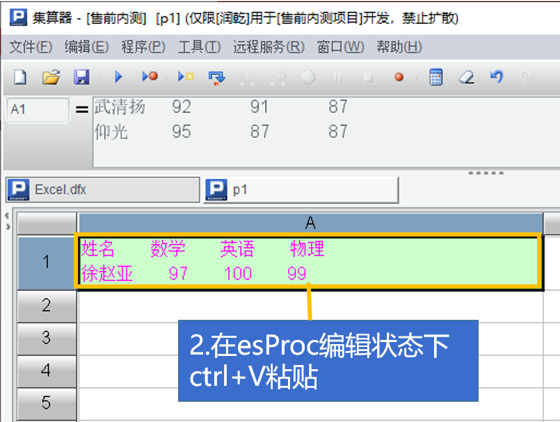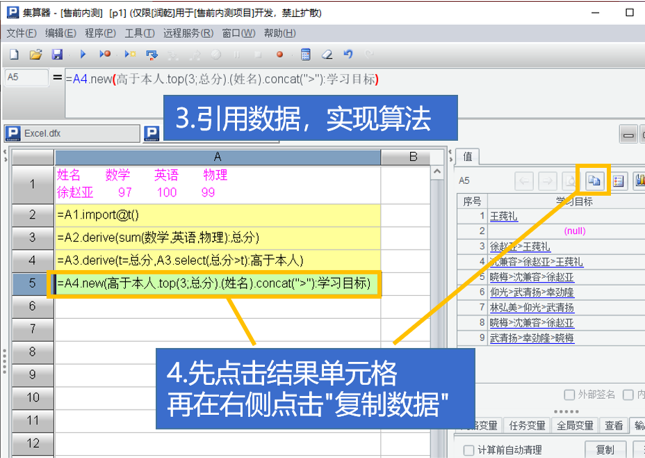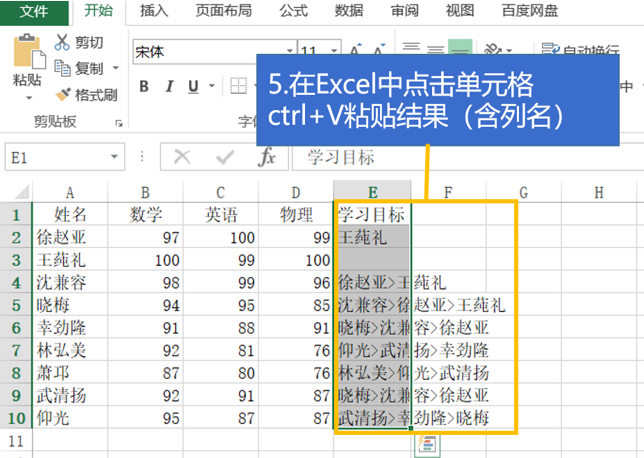#### Excel加载SPL插件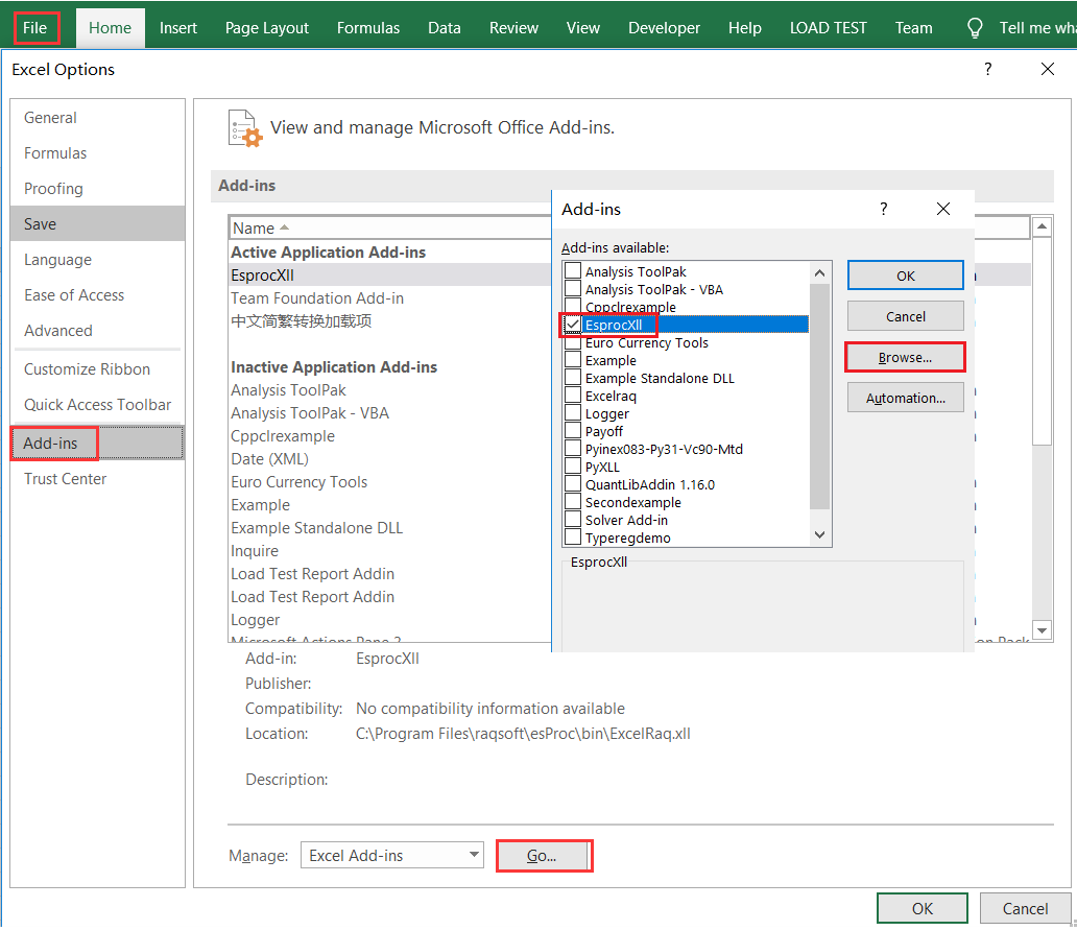#### Excel中使用SPL函数实施计算#### VBA中调用SPL脚本完成计算### 报表工具配合

SPL可以调用报表工具生成、输出报表

#### 1、Excel中使用SPL函数实施计算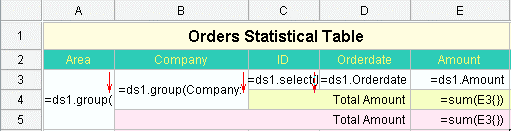#### 2、SPL脚本，调用报表模板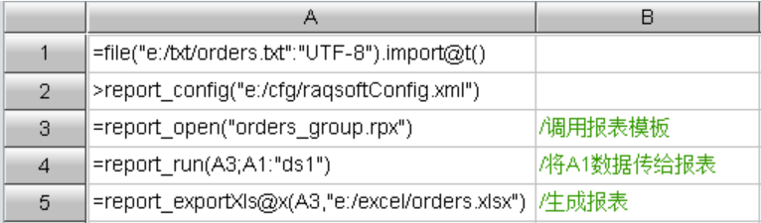#### 3、根据报表模板样式生成的报表（Excel）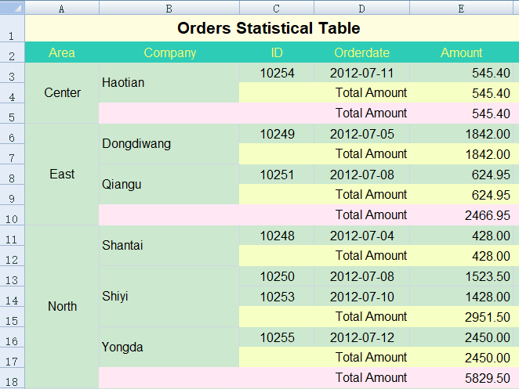### 大数据计算

SPL提供了外存游标、并行计算等机制实现大数据文件计算

Python未提供大数据支持，需要手写代码，非常繁琐

#### 计算目标：将大文件按照订单编号排序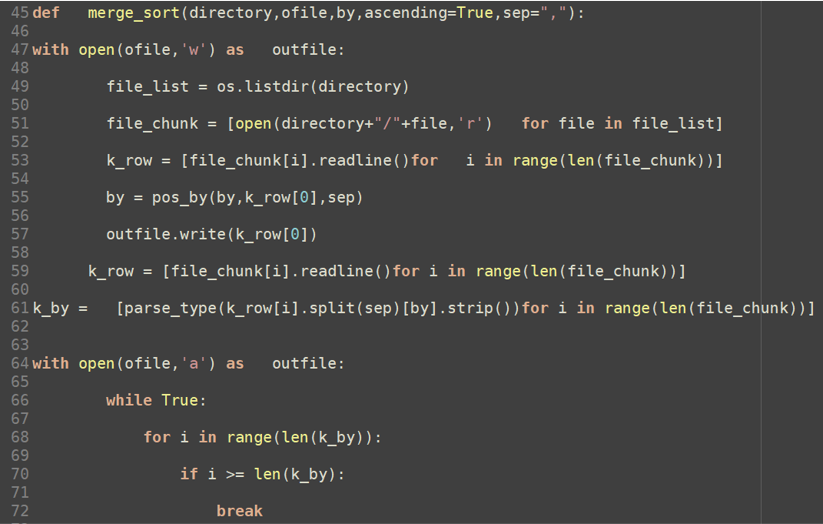##### 部分Python代码（共67行）
A B
1 =file(file_path).cursor@tc() /创建大文件游标
2 =A1.sortx(key) /游标排序
3 =file(out_file).export@tc(A2) /结果流式输出到文件

### 文件SQL查询

#### 计算目标：将大文件按照订单编号排序

##### Students_scores.txt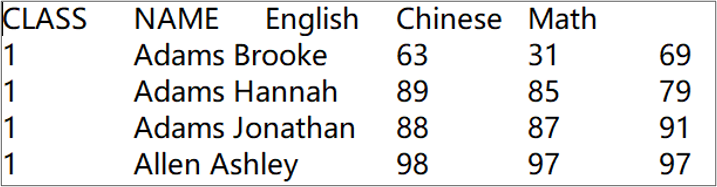##### 分组汇总
A
1 \$select   CLASS,min(English),max(Chinese),sum(Math) from E:/txt/students_scores.txt group by CLASS
##### 关联查询
A
1 \$select   sum(S.quantity*P.Price) as totalfrom E:/txt/Sales.txt as S   join E:/txt/Products.txt as P on S.productid=P.ID where S.quantity<=10